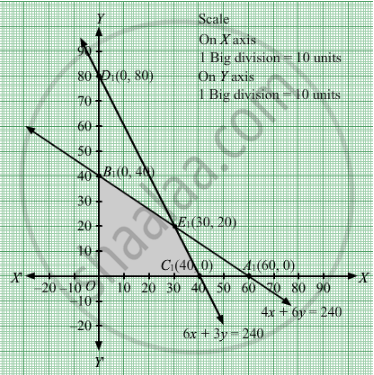# A Factory Manufactures Two Types of Screws, a and B, Each Type Requiring the Use of Two Machines - an Automatic and a Hand-operated. - Mathematics

Sum

A factory manufactures two types of screws, A and B, each type requiring the use of two machines - an automatic and a hand-operated. It takes 4 minute on the automatic and 6 minutes on the hand-operated machines to manufacture a package of screws 'A', while it takes 6 minutes on the automatic and 3 minutes on the hand-operated machine to manufacture a package of screws 'B'. Each machine is available for at most 4 hours on any day. The manufacturer can sell a package of screws 'A' at a profit of 70 P and screws 'B' at a profit of Rs 1. Assuming that he can sell all the screws he can manufacture, how many packages of each type should the factory owner produce in a day in order to maximize his profit? Determine the maximum profit.

#### Solution

Let the factory manufacture x screws of type A and y screws of type B on each day. Therefore,

≥ 0 and ≥ 0

The given information can be compiled in a table as follows.

 Screw A Screw B Availability Automatic Machine (min) 4 6 4 × 60 = 240 Hand Operated Machine (min) 6 3 4 × 60 = 240

$4x + 6y \geq 240$

$6x + 3y \geq 240$

The manufacturer can sell a package of screws 'A' at a profit of Rs 0.7 and screws 'B' at a profit of Re 1.

Total profit, Z = 0.7x + 1y

The mathematical formulation of the given problem is

Maximize Z = 0.7x + 1y

subject to the constraints,

$4x + 6y \geq 240$
$6x + 3y \geq 240$

x, y ≥ 0

First we will convert inequations into equations as follows:
4x + 6y = 240, 6x + 3y = 240, x = 0 and y = 0

Region represented by 4x + 6y ≤ 240:
The line 4x + 6y = 240 meets the coordinate axes at A1(60, 0) and B1(0, 40) respectively. By joining these points we obtain the line 4x + 6y = 240. Clearly (0,0) satisfies the 4x + 6y = 240. So,the region which contains the origin represents the solution set of the inequation 4x + 6y ≤ 240.

Region represented by 6x + 3y ≤ 240:
The line 6x + 3y = 240 meets the coordinate axes at C1(40, 0) and D1(0, 80) respectively. By joining these points we obtain the line 6x + 3y = 240.  Clearly (0,0) satisfies the inequation 6x + 3y ≤ 240. So,the region which contains the origin represents the solution set of the inequation 6x + 3y ≤ 240.

Region represented by x ≥ 0 and y ≥ 0:
Since, every point in the first quadrant satisfies these inequations. So, the first quadrant is the region represented by the inequations x ≥ 0, and ≥ 0.

The feasible region determined by the system of constraints 4x + 6y ≤ 240, 6x + 3y ≤ 240, x ≥ 0, and y ≥ 0 are as follows.The corner points are C1(40, 0), E1(30, 20) and B1(0, 40).

The values of Z at these corner points are as follows.

 Corner point Z = 7x + 10y C1(40, 0) 280 E1(30, 20) 410 B1(0, 40) 400

The maximum value of Z is 410 at (30, 20).

Thus, the factory should produce 30 packages of screws A and 20 packages of screws B to get the maximum profit of Rs 410.

Concept: Graphical Method of Solving Linear Programming Problems
Is there an error in this question or solution?

#### APPEARS IN

RD Sharma Class 12 Maths
Chapter 30 Linear programming
Exercise 30.4 | Q 4 | Page 50